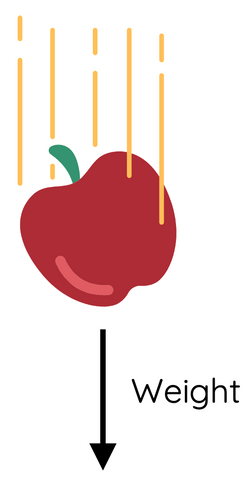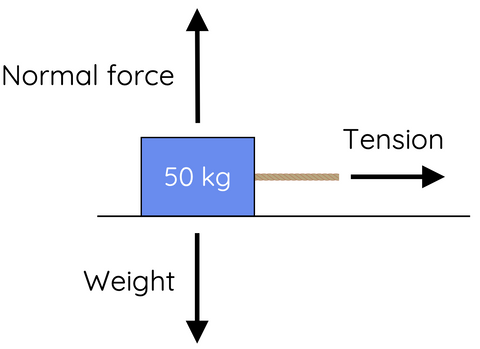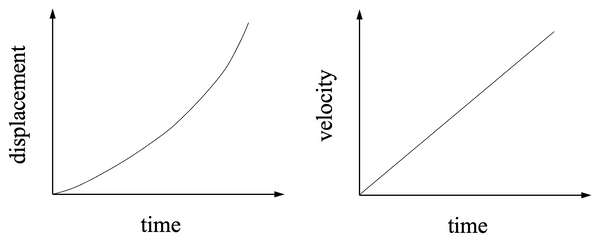# Motion with Constant Force

This topic is part of the HSC Physics course under the section Forces, Acceleration and Energy.

### HSC Physics Syllabus

• investigate, describe and analyse the acceleration of a single object subjected to a constant net force and relate the motion of the object to Newton’s Second Law of Motion through the use of: (ACSPH062, ACSPH063)
– qualitative descriptions
– graphs and vectors
– deriving relationships from graphical representations including 𝐹net = 𝑚𝑎 and relationships of uniformly accelerated motion

### Motion with Constant Force

As HSC Physics students, it's essential to grasp the fundamental concepts of motion with constant force and acceleration. This knowledge forms the basis for understanding the physics of everyday phenomena and the behaviour of objects under various conditions. In this article, we will dive into the key concepts and equations that govern motion with constant force and acceleration.

### Newton's Second Law

The foundation of motion with constant force and acceleration lies in Newton's second law of motion, which states:

$$F=ma$$

Where F is the net force acting on an object, m is the mass of the object, and a is the acceleration of the object. According to this law, when a constant net force is applied to an object with constant mass, it experiences constant acceleration.

### Constant Force and Acceleration

When an object experiences a constant force, it undergoes a constant acceleration, as described by Newton's second law. This means that the object's velocity changes at a constant rate over time. The relationship between displacement (s), initial velocity (u), final velocity (v), acceleration (a), and time (t) can be described by the following three equations of motion:

$$v = u + at$$

$$s = ut + \frac{1}{2}at^2$$

$$v^2 = u^2 + 2as$$

These equations, also known as the kinematic equations, can be used to analyse the motion of an object under constant force and acceleration.

Read more about motion with constant acceleration in the Kinematics Module here

### Practical Examples of Motion with Constant Force

Let's explore two practical examples to understand the application of the concepts discussed above:Free Fall: When an object is in free fall near the Earth's surface, it experiences a constant acceleration due to gravity (g ≈ 9.81 m/s²). In this case, the net force acting on the object is the gravitational force (F_g = mg). This scenario assumes the absence of air resistance e.g. in space.

Horizontal Motion with Air Resistance Neglected: When an object moves horizontally with constant force, such as a pushed object on a frictionless surface, it experiences constant acceleration in the direction of the applied force.

Consider a mass being pulled with a tension force horizontally along a frictionless surface.In the vertical direction, the normal force exerted by the surface balances the mass's weight force. In the horizontal force, the net force equals the tension force.

Rocket Propulsion

Consider a rocket that is launched vertically with a propulsion system generating constant thrust (F_T).During the rocket's ascent, there are two primary forces acting upon it:

• Weight force, F_g=mg directed vertically downward
• Thrust (F_T) directed vertically upward

As the rocket ascends, its fuel is continuously consumed, leading to a decrease in mass. Consequently, the rocket's mass changes over time. However, for simplicity, we will assume that the rocket's thrust remains constant despite this change in mass.

According to Newton's second law, the net force acting on the rocket is given by:

$$F_{net} = F_T - F_g$$

$$F_{net} = F_T - mg$$

The net force determines the rocket's acceleration:

$$a = \frac{F_{net}}{m}$$

$$a = \frac{(F_T - mg)}{m}$$

Since the thrust (T) is constant, the acceleration of the rocket increases as its mass decreases due to fuel consumption. This example demonstrates that a constant net force only produces constant acceleration if the object's mass remains constant.

### Graphical RepresentationThe motion of an object under constant force and acceleration can also be represented graphically:

Displacement-Time (s-t) Graph: A parabolic curve, with the equation

$$s = ut + \frac{1}{2}at^2$$

Velocity-Time (v-t) Graph: A linear graph, with the equation

$$v = u + at$$

The slope of the s-t graph represents the velocity of the object, while the slope of the v-t graph represents the acceleration. The area under the v-t graph corresponds to the displacement of the object.